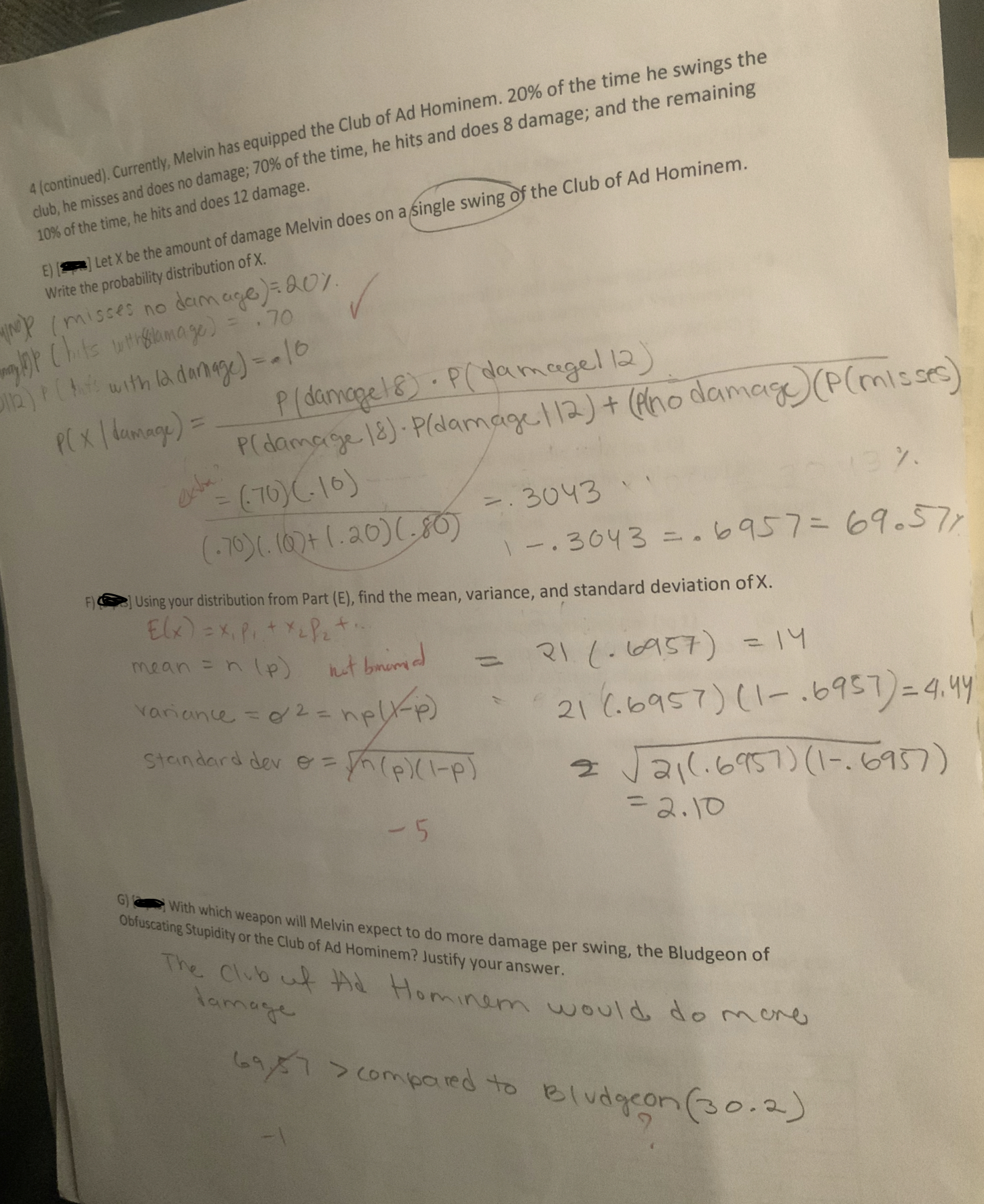# 4 (continued). Currently, Melvin has equipped the Club of Ad Hominem. 20% of the time he swings theclub, he misses and does no damage,70% of the time, he hits and does 8 damage; and the remaining10% of the time, he hits and does 12 damage.ELet X be the amount of damage Melvin does on a Single swing of the Club of Ad HWrite the probability distribution of X.Cimc304336437677Using your distribution from Part (E), find the mean, variance, and standard deviation of X.stanaard der eh(PIPG With which weapon will Melvin expect to do more damage per swing, the Bludgeon ofObfuscating Stupidity or the Club of Ad Hominem? Justify your answerThe Clubtt Hominam would do r-lameconmieied to el o o

Question
18 viewshelp_outlineImage Transcriptionclose4 (continued). Currently, Melvin has equipped the Club of Ad Hominem. 20% of the time he swings the club, he misses and does no damage,70% of the time, he hits and does 8 damage; and the remaining 10% of the time, he hits and does 12 damage. ELet X be the amount of damage Melvin does on a Single swing of the Club of Ad H Write the probability distribution of X. Cimc 3043 36437677 Using your distribution from Part (E), find the mean, variance, and standard deviation of X. stanaard der eh(PIP G With which weapon will Melvin expect to do more damage per swing, the Bludgeon of Obfuscating Stupidity or the Club of Ad Hominem? Justify your answer The Club tt Hominam would do r- lame conmieied to el o o fullscreen
check_circle

Step 1

The given information is not sufficient to solve all the subparts. More information is required to solve Part (G). So, we will answer first two subparts.

Step 2

E) Obtaining the probability distribution of X:

It was found that 20% of the time, Melvin misses and does no damage, 70% of the time he hits and does 8 damage and 10% of the time he hits and does 12 damage.

The random variable X denotes the amount of damage Melvin does in a single swing.

That is, the random variable X takes values 0, 8 and 12 with probabilities 0.20(=20%), 0.70(=70%) and 0.10(=10%) respectively.

The probability distribution of a random variable X is the set of all possible values of the random variable X and its corresponding probabilities.

From the given information, the probability distribution of X is obtained and is given in the following table:

Step 3

F) Obtaining the mean, variance and standard deviation of X:

The mean value of a discrete random variable X is given by,

E(X) = µx = ∑x.P(...

### Want to see the full answer?

See Solution

#### Want to see this answer and more?

Solutions are written by subject experts who are available 24/7. Questions are typically answered within 1 hour.*

See Solution
*Response times may vary by subject and question.
Tagged in

### Other